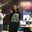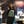Trusted answers to developer questions
Trusted Answers to Developer Questions

Related Tags

nfa
dfa
theoretical cs

# How to convert NFA to DFAAyesha Kanwal

Grokking Modern System Design Interview for Engineers & Managers

Ace your System Design Interview and take your career to the next level. Learn to handle the design of applications like Netflix, Quora, Facebook, Uber, and many more in a 45-min interview. Learn the RESHADED framework for architecting web-scale applications by determining requirements, constraints, and assumptions before diving into a step-by-step design process.

### NFA vs. DFA

A deterministic finite automaton has one transition from one state to another. On the other hand, a nondeterministic finite automaton has one or more than one transition from one state to another or itself.

Every deterministic finite automaton is a nondeterministic finite automaton but the reverse is not true.

### Subset construction method

Assume an NFA for a language L with $$ where

• $Q$ is the finite set of states
• $Σ$ is the input symbols
• $q_0$ is the start state
• $δ$ is the transition function
• $F$ is the final state

It is converted to a DFA $$ where

• $Q'$ is the new finite set of states
• $Σ$ is the input symbols
• $q_0$ is the start state
• $δ'$ is the transition function
• $F'$ is the new final state

Note: While converting an NFA with n states to a DFA, 2n possible set of states can be reachable but not necessarily reached in the DFA.

The following general set of rules are followed to convert a nondeterministic finite automaton to a deterministic finite automaton:

1. At the beginning $Q'$ = $∅$.
2. Add $q_0$ to $Q'$.
3. For every state in $Q'$, find the possible set of states for each input symbol using the transition function of the NFA. If the acquired set of states is not present in $Q'$, add it.
4. The final state $F'$ will be all those states that have $F$ in them.

### Example

Let's consider the following nondeterministic finite automaton that accepts the language $\{{aa, aab}\}^*\{{b}\}$.

For this NFA, $q0$ is the start state.

NFA

δ = Transition Table
 state a b q0 q1, q2 q4 q1 q0 - q2 q3 - q3 - q0 q4 - -

#### Step 1: Q' is empty

Checks if $Q'$ is empty.

#### Step 2: Add the first state to Q'

Add the first state to $Q'$ such that it now has $q_0$.

#### Step 3: Find states for each input symbol

For each state in $Q'$, we will find the states for each of the input symbols. $Q'$ has $q_0$ presently. Check transitions for '$a$' and '$b$' from the transition table for $q_0$. Update the table as new states are reached.

##### Step 3.1:
Transition Table for q0
 state a b q0 q1, q2 q4
##### Step 3.2:
Transition Table for {q1,q2} and {q4}
 state a b q0 q1, q2 q4 q1, q2 q0, q3 - q4 - -
##### Step 3.3:
Transition Table for {q0,q3}
 state a b q0 q1, q2 q4 q1, q2 q0, q3 - q4 - - q0, q3 q1 q0, q4
##### Step 3.4:
Transition Table for {q1} and {q0, q4}
 state a b q0 q1, q2 q4 q1, q2 q0, q3 - q4 - - q0, q3 q1, q2 q4 q1 q0 - q0, q4 q1, q2 q4

#### Step 4: Result

Combine all the states in the last obtained transition table and show appropriate transitions. In case a transition is not defined for input, make a transition to the trap state.

For this DFA, $q0$ is the start state.

DFA

RELATED TAGS

nfa
dfa
theoretical cs

CONTRIBUTORAyesha Kanwal